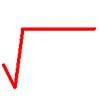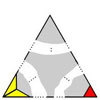# Resources tagged with: Mathematical reasoning & proof

Filter by: Content type:
Age range:
Challenge level:

### There are 174 results

Broad Topics > Using, Applying and Reasoning about Mathematics > Mathematical reasoning & proof### The Clue Is in the Question

##### Age 16 to 18 Challenge Level:

Starting with one of the mini-challenges, how many of the other mini-challenges will you invent for yourself?### To Prove or Not to Prove

##### Age 14 to 18

A serious but easily readable discussion of proof in mathematics with some amusing stories and some interesting examples.### Rational Roots

##### Age 16 to 18 Challenge Level:

Given that a, b and c are natural numbers show that if sqrt a+sqrt b is rational then it is a natural number. Extend this to 3 variables.### No Right Angle Here

##### Age 14 to 16 Challenge Level:

Prove that the internal angle bisectors of a triangle will never be perpendicular to each other.### Proof of Pick's Theorem

##### Age 16 to 18 Challenge Level:

Follow the hints and prove Pick's Theorem.### The Root Cause

##### Age 16 to 18 Challenge Level:

Prove that if a is a natural number and the square root of a is rational, then it is a square number (an integer n^2 for some integer n.)### Janine's Conjecture

##### Age 14 to 16 Challenge Level:

Janine noticed, while studying some cube numbers, that if you take three consecutive whole numbers and multiply them together and then add the middle number of the three, you get the middle number. . . .### Sperner's Lemma

##### Age 16 to 18

An article about the strategy for playing The Triangle Game which appears on the NRICH site. It contains a simple lemma about labelling a grid of equilateral triangles within a triangular frame.### Multiplication Square

##### Age 14 to 16 Challenge Level:

Pick a square within a multiplication square and add the numbers on each diagonal. What do you notice?### Iffy Logic

##### Age 14 to 18 Challenge Level:

Can you rearrange the cards to make a series of correct mathematical statements?### Contrary Logic

##### Age 16 to 18 Challenge Level:

Can you invert the logic to prove these statements?### Triangular Intersection

##### Age 14 to 16 Short Challenge Level:

What is the largest number of intersection points that a triangle and a quadrilateral can have?### On the Importance of Pedantry

##### Age 16 to 18

A introduction to how patterns can be deceiving, and what is and is not a proof.### More Number Sandwiches

##### Age 11 to 16 Challenge Level:

When is it impossible to make number sandwiches?### Dodgy Proofs

##### Age 16 to 18 Challenge Level:

These proofs are wrong. Can you see why?##### Age 16 to 18 Short Challenge Level:

Can you work out where the blue-and-red brick roads end?### Diophantine N-tuples

##### Age 14 to 16 Challenge Level:

Can you explain why a sequence of operations always gives you perfect squares?### Mouhefanggai

##### Age 14 to 16

Imagine two identical cylindrical pipes meeting at right angles and think about the shape of the space which belongs to both pipes. Early Chinese mathematicians call this shape the mouhefanggai.### Water Pistols

##### Age 16 to 18 Challenge Level:

With n people anywhere in a field each shoots a water pistol at the nearest person. In general who gets wet? What difference does it make if n is odd or even?### Proof Sorter - Quadratic Equation

##### Age 14 to 18 Challenge Level:

This is an interactivity in which you have to sort the steps in the completion of the square into the correct order to prove the formula for the solutions of quadratic equations.### Dalmatians

##### Age 14 to 18 Challenge Level:

Investigate the sequences obtained by starting with any positive 2 digit number (10a+b) and repeatedly using the rule 10a+b maps to 10b-a to get the next number in the sequence.### Converse

##### Age 14 to 16 Challenge Level:

Clearly if a, b and c are the lengths of the sides of an equilateral triangle then a^2 + b^2 + c^2 = ab + bc + ca. Is the converse true?### Pareq Exists

##### Age 14 to 16 Challenge Level:

Prove that, given any three parallel lines, an equilateral triangle always exists with one vertex on each of the three lines.### Calculating with Cosines

##### Age 14 to 18 Challenge Level:

If I tell you two sides of a right-angled triangle, you can easily work out the third. But what if the angle between the two sides is not a right angle?### Russian Cubes

##### Age 14 to 16 Challenge Level:

I want some cubes painted with three blue faces and three red faces. How many different cubes can be painted like that?### Proof: A Brief Historical Survey

##### Age 14 to 18

If you think that mathematical proof is really clearcut and universal then you should read this article.### Polynomial Relations

##### Age 16 to 18 Challenge Level:

Given any two polynomials in a single variable it is always possible to eliminate the variable and obtain a formula showing the relationship between the two polynomials. Try this one.### Proofs with Pictures

##### Age 14 to 18

Some diagrammatic 'proofs' of algebraic identities and inequalities.### Mechanical Integration

##### Age 16 to 18 Challenge Level:

To find the integral of a polynomial, evaluate it at some special points and add multiples of these values.### Impossible Sandwiches

##### Age 11 to 18

In this 7-sandwich: 7 1 3 1 6 4 3 5 7 2 4 6 2 5 there are 7 numbers between the 7s, 6 between the 6s etc. The article shows which values of n can make n-sandwiches and which cannot.### Cosines Rule

##### Age 14 to 16 Challenge Level:

Three points A, B and C lie in this order on a line, and P is any point in the plane. Use the Cosine Rule to prove the following statement.### Square Mean

##### Age 14 to 16 Challenge Level:

Is the mean of the squares of two numbers greater than, or less than, the square of their means?### There's a Limit

##### Age 14 to 18 Challenge Level:

Explore the continued fraction: 2+3/(2+3/(2+3/2+...)) What do you notice when successive terms are taken? What happens to the terms if the fraction goes on indefinitely?### Transitivity

##### Age 16 to 18

Suppose A always beats B and B always beats C, then would you expect A to beat C? Not always! What seems obvious is not always true. Results always need to be proved in mathematics.### Magic Squares II

##### Age 14 to 18

An article which gives an account of some properties of magic squares.### Where Do We Get Our Feet Wet?

##### Age 16 to 18

Professor Korner has generously supported school mathematics for more than 30 years and has been a good friend to NRICH since it started.### Can it Be

##### Age 16 to 18 Challenge Level:### Pair Squares

##### Age 16 to 18 Challenge Level:

The sum of any two of the numbers 2, 34 and 47 is a perfect square. Choose three square numbers and find sets of three integers with this property. Generalise to four integers.### Postage

##### Age 14 to 16 Challenge Level:

The country Sixtania prints postage stamps with only three values 6 lucres, 10 lucres and 15 lucres (where the currency is in lucres).Which values cannot be made up with combinations of these postage. . . .### An Alphanumeric

##### Age 16 to 18

Freddie Manners, of Packwood Haugh School in Shropshire solved an alphanumeric without using the extra information supplied and this article explains his reasoning.### Impossible Triangles?

##### Age 16 to 18 Challenge Level:

Which of these triangular jigsaws are impossible to finish?### Same Length

##### Age 11 to 16 Challenge Level:

Construct two equilateral triangles on a straight line. There are two lengths that look the same - can you prove it?### The Great Weights Puzzle

##### Age 14 to 16 Challenge Level:

You have twelve weights, one of which is different from the rest. Using just 3 weighings, can you identify which weight is the odd one out, and whether it is heavier or lighter than the rest?### Diverging

##### Age 16 to 18 Challenge Level:

Show that for natural numbers x and y if x/y > 1 then x/y>(x+1)/(y+1}>1. Hence prove that the product for i=1 to n of [(2i)/(2i-1)] tends to infinity as n tends to infinity.### Tetra Inequalities

##### Age 16 to 18 Challenge Level:

Prove that in every tetrahedron there is a vertex such that the three edges meeting there have lengths which could be the sides of a triangle.##### Age 14 to 18 Challenge Level:

Which of these roads will satisfy a Munchkin builder?### A Long Time at the Till

##### Age 14 to 18 Challenge Level:

Try to solve this very difficult problem and then study our two suggested solutions. How would you use your knowledge to try to solve variants on the original problem?### Advent Calendar 2011 - Secondary

##### Age 11 to 18 Challenge Level:

Advent Calendar 2011 - a mathematical activity for each day during the run-up to Christmas.### Notty Logic

##### Age 16 to 18 Challenge Level:

Have a go at being mathematically negative, by negating these statements.### Zig Zag

##### Age 14 to 16 Challenge Level:

Four identical right angled triangles are drawn on the sides of a square. Two face out, two face in. Why do the four vertices marked with dots lie on one line?